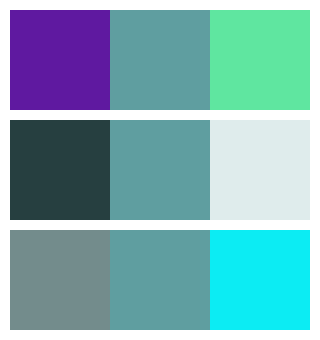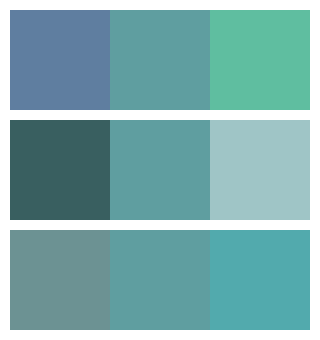# Derived colour in generativepy

Martin McBride, 2021-11-10
Tags generativepy tutorial colour derived colour
Categories generativepy generativepy tutorialIf you have an existing colour, you can create a new derived colour based on the existing colour. There are two ways of doing this:

• Replace one colour value (for example the red value) with a new value, leaving the other colours the same.
• Multiply one colour value (for example the red value) by a factor, leaving the other colours the same.

You can apply these changes to the RGB value, the alpha values, or the HSL values.

## Colour channel replacement

When we set a new, replacement value for one of the channels, then that channel alone will change. For example, this code creates a new colour with its r value set to 0.5 (it leaves the g, b and alpha values unchanged):

col_new = col.with_r(0.5)


Similar functions exist for g (green), b (blue), a (alpha), h (hue), s (saturation), and l (lightness). You can apply these functions to any colour. For example, even if a colour was initially defined with RGB values, you can still alter its saturation. Under the hood, the colour will be converted to HSL, modified, then converted back to RGB.

The replacement value will be clamped to the range 0.0 to 1.0. Here is some example code:

from generativepy.drawing import make_image, setup
from generativepy.color import Color
from generativepy.geometry import Rectangle

def draw_with(ctx, pixel_width, pixel_height, frame_no, frame_count):
setup(ctx, pixel_width, pixel_height, background=Color(1))

pos = [10, 10]
w = 100
h = 100

Rectangle(ctx).of_corner_size(pos, w, h).fill(col.with_g(0.1))
pos += w
Rectangle(ctx).of_corner_size(pos, w, h).fill(col)
pos += w
Rectangle(ctx).of_corner_size(pos, w, h).fill(col.with_g(0.9))

pos = [10, 120]

Rectangle(ctx).of_corner_size(pos, w, h).fill(col.with_l(0.2))
pos += w
Rectangle(ctx).of_corner_size(pos, w, h).fill(col)
pos += w
Rectangle(ctx).of_corner_size(pos, w, h).fill(col.with_l(0.9))

pos = [10, 230]

Rectangle(ctx).of_corner_size(pos, w, h).fill(col.with_s(0.1))
pos += w
Rectangle(ctx).of_corner_size(pos, w, h).fill(col)
pos += w
Rectangle(ctx).of_corner_size(pos, w, h).fill(col.with_s(0.9))

make_image("colour-with.png", draw_with, 320, 340)


This code is available on github in tutorial/colour/colour_with.py.

Here is the image it creates:The image uses a base colour of cadetblue.

The first line of 3 colours shows the colour cadetblue - with green set to 0.1, unmodified, and with green set to 0.9.

The second line shows the same colour - with lightness set to 0.2, unmodified, and with lightness set to 0.9.

The third line shows the same colour - with saturation set to 0.1, unmodified, and with saturation set to 0/9.

## Colour channel factor

This code creates a new colour with its r value multiplied by 1.2:

col_new = col.with_r_factor(1.2)


As with the with_r method, similar functions exist for g, b, h, s, l and a.

You can use a factor that is greater than 1 to increase the amount of red in the colour, or a factor that is less than 1 to decrease the amount of red in the colour. For example, if the existing value of red was 0.5 and you apply a factor of 1.2, the new red value is 0.6. Bear in mind:

• If the colour has no red to start with (for instance the RGB colour (0, 1, 0.3), then with_r_factor will have no effect because it is just multiplying 0 by a factor. You can still use with_r to add red into a colour.
• The total amount of red in the colour is clamped at the maximum, 1.0. Once you hit the limit then increasing the factor won't make the colour any redder.

This code illustrates the use of with_g_factor, with_l_factor, and with_s_factor:

from generativepy.drawing import make_image, setup
from generativepy.color import Color
from generativepy.geometry import Rectangle

def draw_delta(ctx, pixel_width, pixel_height, frame_no, frame_count):
setup(ctx, pixel_width, pixel_height, background=Color(1))

pos = [10, 10]
w = 100
h = 100

Rectangle(ctx).of_corner_size(pos, w, h).fill(col.with_g_factor(0.8))
pos += w
Rectangle(ctx).of_corner_size(pos, w, h).fill(col)
pos += w
Rectangle(ctx).of_corner_size(pos, w, h).fill(col.with_g_factor(1.2))

pos = [10, 120]

Rectangle(ctx).of_corner_size(pos, w, h).fill(col.with_l_factor(0.6))
pos += w
Rectangle(ctx).of_corner_size(pos, w, h).fill(col)
pos += w
Rectangle(ctx).of_corner_size(pos, w, h).fill(col.with_l_factor(1.4))

pos = [10, 230]

Rectangle(ctx).of_corner_size(pos, w, h).fill(col.with_s_factor(0.6))
pos += w
Rectangle(ctx).of_corner_size(pos, w, h).fill(col)
pos += w
Rectangle(ctx).of_corner_size(pos, w, h).fill(col.with_s_factor(1.4))

make_image("colour-delta.png", draw_delta, 320, 340)


This code is available on github in tutorial/colour/colour_delta.py.

Here is the image it creates:The first line of 3 colours shows the colour cadetblue - with green reduced to 80%, unmodified, and with green increased to 120%.

The second line shows the same colour - with lightness reduced to 60%, unmodified, and with lightness increased to 140%.

The third line shows the same colour - with saturation reduced to 60%, unmodified, and with saturation increased to 140%.

If you found this article useful, you might be interested in the book Computer Graphics in Python or other books by the same author.

#### Popular tags

2d arrays abstract data type alignment and angle animation arange arc array arrays behavioural pattern bezier curve built-in function callable object chain circle classes clipping close closure cmyk colour combinations comparison operator comprehension context context manager conversion count creational pattern data science data types design pattern device space dictionary drawing duck typing efficiency ellipse else encryption enumerate fill filter font font style for loop function function composition function plot functools game development generativepy tutorial generator geometry gif global variable gradient greyscale higher order function hsl html image image processing imagesurface immutable object in operator index inner function input installing iter iterable iterator itertools join l system lambda function len line linear gradient linspace list list comprehension logical operator lru_cache magic method mandelbrot mandelbrot set map matplotlib monad mutability named parameter numeric python numpy object open operator optimisation optional parameter or pandas partial application path pattern permutations polygon positional parameter print pure function python standard library radial gradient range recipes rectangle recursion reduce repeat rgb rotation roundrect scaling scipy sector segment sequence setup shape singleton slice slicing sound spirograph sprite square str stream string stroke structural pattern subpath symmetric encryption template text text metrics tinkerbell fractal transform translation transparency triangle truthy value tuple turtle unpacking user space vectorisation webserver website while loop zip